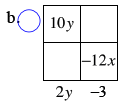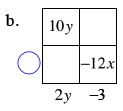Home > CCA > Chapter 5 > Lesson 5.3.1 > Problem5-89

5-89.

Find the missing areas and dimensions for each generic rectangle below. Then write each area as a sum and as a product. Homework Help ✎

1.  $4x$ $16x^2$ $1$
• (4x + 1)(4x + 1) = 16x2 + 8x +1

1.  $10y$ $-12y$ $2y$ $-3$
• What must be multiplied by 2y to get 10y? Put that expression where the blue circle is.

•• What must be multiplied by −3 to get −12x? Put that expression where the blue circle is.

•